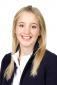# Overlap of input matrices (missing variables)

5 posts / 0 newOffline
Joined: 08/22/2016 - 03:14
Overlap of input matrices (missing variables)

Hello,

I am Valerie, 25 years old and absolutely fascinated by applying Metasem respectively TSSEM by using metaSEM. When I was doing my first analysis, I came across a problem concerning the input data respectively the input correlation matrices and did not find an solution so far:

Let's say, I have two studies, which are dedicated to the same topic, but slightly differ in their observed variables, what is also reflected in their correlation matrices.
1) Study1 shows a correlation matrix with the variables x1,x2,x3
2) Study2 shows a corrleation matrix with the variables x1,x2,x4
This data is different when compared to the datasets such as Hunter83 provided in the book (Cheung 2015), because in this case, there is no correlation matrix, which is "complete", as variables (and as a result also the correlations) are missing in the studies.
Then input matrix would look as follows (taking random values for correlation coefficients):
1
0.2 1
0.3 0.5 1
NA NA NA NA
1
0.7 1
NA NA NA
0.4 0.2 NA 1

When I imported the data using the readLowMat() function, it worked out. However, when applying tssem1(), there is an error message in case of method="FEM" (Error in if (!all(isPD)) warning(paste("Group ", (1:no.groups)[!isPD], :
missing value where TRUE/FALSE needed) and in case of method="REM" (Error in if (all.equal(covMatrix, t(covMatrix))) { :
argument is not interpretable as logical). My guess was that the kind of input data is not suitable for TSSEM as there is not "complete matrix" available, when it comes to the equality constraints. But I do not the correct procedure to apply now. I have read about pairwise or listwise deletion, but isn't this an issue, TSSEM is able to avoid? Or do I mix up the cases of missing variables and missign correlations?

I really would appreciate any help and good advice. I send best wishes from Germany!Offline
Joined: 10/08/2009 - 22:37
Hi Valerie, It is a

Hi Valerie,

It is a misconception that a study with complete data is required by TSSEM. The attached example shows that it works fine even when there is no complete data.

In your example, there is no correlation between variables 3 and 4. We should expect that it does not work. If it works, it is a bug!

Best,
Mike

library(metaSEM)

mat1 <- create.mxMatrix(c(1,
0.2, 1,
0.3, 0.5, 1,
NA, NA, NA, NA), type="Symm",
ncol=4, nrow=4, byrow=TRUE, as.mxMatrix = FALSE)

mat2 <- create.mxMatrix(c(1,
0.7, 1,
NA, NA, NA,
0.4, 0.2, NA, 1), type="Symm",
ncol=4, nrow=4, byrow=TRUE, as.mxMatrix = FALSE)

my.df1 <- list(mat1, mat2)

pattern.na(my.df1, show.na=FALSE)

## It returns an error as there is no correlation for variables 4 and 3.

fixed1 <- tssem1(my.df1, n=c(100,100), method = "FEM")

mat3 <- create.mxMatrix(c(1,
NA, NA,
0.4, NA, 1,
0.4, NA, 0.3, 1), type="Symm",
ncol=4, nrow=4, byrow=TRUE, as.mxMatrix = FALSE)

my.df2 <- list(mat1, mat2, mat3)

pattern.na(my.df2, show.na=FALSE)

## It works fine even there is no complete study.

fixed2 <- tssem1(my.df2, n=c(100,100,100), method = "FEM")
summary(fixed2)Offline
Joined: 08/22/2016 - 03:14
Thank you

Hi Mike,

thank you very much, this helps a lot!

Best wishesOffline
Joined: 08/22/2016 - 03:14
Hi Mike,just to clarify, I

Hi Mike,

just to clarify, I need to ask one more question:
In your example your created mat3. So mat1, mat2, and mat3 complement each other in a way that there is never a missing correlation in the pooled matrix.

This is not the case in my real-world example. There are still gaps which are not complemented. So do you know how to solve the problem? I couldn't find any literature in this case.

Thank you so much.
ValerieOffline
Joined: 10/08/2009 - 22:37
Hi Valerie, It seems that you

Hi Valerie,

It seems that you are referring to the case that there is no data at all for a particular correlation coefficient. If there is no data in a meta-analysis, there is not much we can do. My suggestion is to simplify the model by excluding the relevant variables.

Best,
Mike